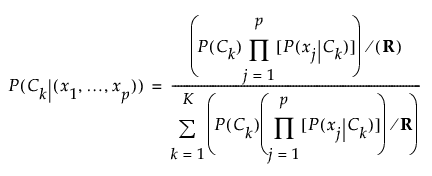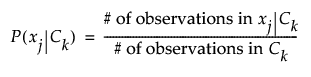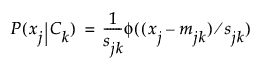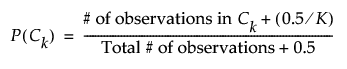Publication date: 05/24/2021

##Algorithm

The naive Bayes method classifies an observation into the class for which its probability of membership, given the values of its features, is highest. The method assumes that the features are conditionally independent within each class.

Denote the possible classifications by C1, …, CK. Denote the features, or predictors, by X1, X2, …, Xp.

The conditional probability that an observation with predictor values x1, x2, …, xp belongs in the class Ck is computed as follows:,

where R is a regularization constant. In the formula above, the conditional probability that an observation with Xj = xj belongs to the class Ck, P(xj|Ck), is determined as follows:

If Xj is categorical:If Xj is continuous:Here, φ is the standard normal density function, and m and s are the mean and standard deviation, respectively, of the predictor values within the class Ck.

The unconditional probability that an observation belongs in class Ck, P(Ck), is computed as follows:An observation is classified into the class for which its conditional probability is the largest.

Note: In the formula for P(Ck), 0.5 is the prior bias factor. This value is the default value. To change the prior default factor, go to File > Preferences > Platforms > Naive Bayes, select the Prior Bias check box and change the value.